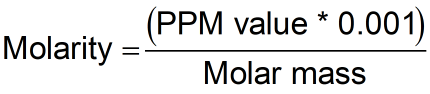Request a Tool

PPM to Molarity Calculator

Molarity are measures of concentration

Molarity
0

Formula• PPM = Parts Per Million

Defination / Uses

The number of "parts" of something in a million "parts" of something else is expressed in parts per million. The "part" can be any unit, although ppm is commonly used to represent weight units when mixing liquids.

Molarity is just like molar concentration. it tells you how many moles of molecules are in a liter of distilled water.

You just need one item to convert ppm to molarity or molarity to ppm the molar mass of the dissolved element or molecule.
You get g/L by multiplying the molarity (in mol/L) by the molar mass (in g/mol). To convert g to mg, multiply g/L by 1000, and you'll get ppm (in mg/L of water).

The following are some examples of when you might need to convert ppm to molarity:

1. The quality of drinking water is measured.
2. Chemical levels in aquariums must be maintained.
3. Fertilizer options for horticulture are mixed.
4. Chemical solutions are created.